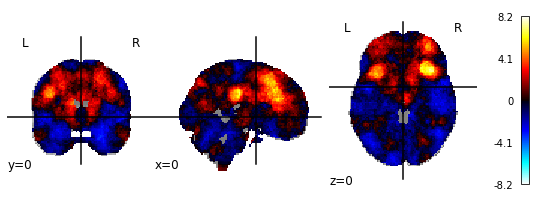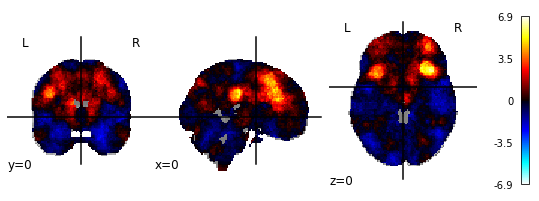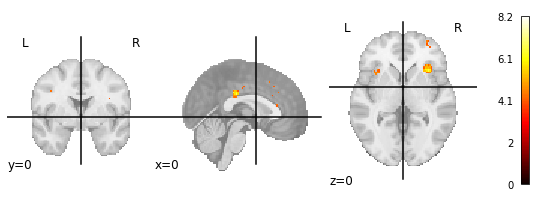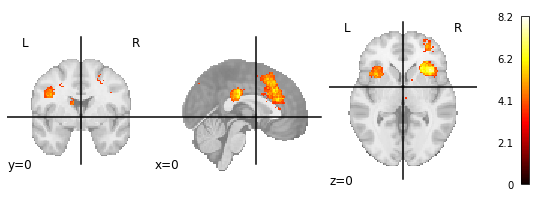# Reproduce association map from Neurosynth with NiMARE

I would like to use NiMARE to create the same association map that one would get when following this neurosynth link and then manually downloading the association map. I was able to implement the following code, which seems to go in the right direction, but it seems that neurosynth.org does one more additional step (cluster-thresholding perhaps?) which leads to a positive-only + thresholded map. How do I have to adapt my script to reproduce this statistical map?

Here’s my code:

``````import nimare
from utils import get_nimare_dataset
from nilearn.plotting import plot_stat_map

###############################################################################
## Analysis settings ###########################´###############################
###############################################################################

# set term of interest
term = 'terms_abstract_tfidf__cognitive control'

# this just gets everything behind the two underscores (for plotting)
term_name = term.split("__")

# get neurosynth data
databases = nimare.extract.fetch_neurosynth(data_dir='../data')

# convert to NiMARE dataset (can take a while so we cache)
ds = nimare.io.convert_neurosynth_to_dataset(
coordinates_file=databases['coordinates'],
annotations_files=databases['features']
)

# get all studies that are associated with the term
term_ids = ds.get_studies_by_label(labels=term,label_threshold=0.001)

# now get all studies that do NOT include this term
all_ids = ds.ids
notterm_ids = sorted(list(set(all_ids) - set(term_ids)))
term_dset = ds.slice(term_ids)
notterm_dset = ds.slice(notterm_ids)

# Run the meta-analysis.
# No multiple comparisons correction since you want the unthreshold maps.
meta = nimare.meta.cbma.mkda.MKDAChi2()
results = meta.fit(term_dset, notterm_dset)
results.save_maps(output_dir="../data/neurosynth_results", prefix=term_name)

# correct for multiple comparisons
corrector = nimare.correct.FDRCorrector(alpha=0.01)
results_corrected = corrector.transform(results)
results_corrected.save_maps(output_dir="../data/neurosynth_results", prefix=term_name)

# plot stat map before multiple comparisons
plot_stat_map(stat_map_img='../data/neurosynth_results/cognitive control_z_desc-specificity.nii.gz',
cut_coords=(0,0,0))

# plot stat map after multiple comparisons
plot_stat_map(stat_map_img='../data/neurosynth_results/cognitive control_z_desc-specificity_level-voxel_corr-FDR_method-indep.nii.gz',
cut_coords=(0,0,0))

# this first by following this link (https://neurosynth.org/analyses/terms/cognitive%20control/)
plot_stat_map(stat_map_img='../data/neurosynth_results/cognitive control_association-test_z_FDR_0.01.nii.gz',
cut_coords=(0,0,0))
``````

Here’s how the statistical maps look like:

1.) NiMARE (without FDR-correction)2.) NiMARE (with FDR-correction)P.S.: In the neurosynth FAQ it says:

The images you see are thresholded to correct for multiple comparisons. We use a false discovery rate (FDR) criterion of .01, meaning that, in theory, and on average, you can expect about 1% of the voxels you see activated in any given map to be false positives (though the actual proportion will vary and is impossible to determine).

But why does the maximum value of 8.2 of the neurosynth-image then matches with the uncorrected image and not the corrected one?

NiMARE corrects the p-values and then generates the z-maps from the corrected p-values. In that sense, the maps are corrected, but they are still unthresholded. On the other hand, the maps in Neurosynth were corrected by applying that threshold. I believe that’s why the maximum values are different.

I think a similar correction could be achieved by applying threshold_stats_img on the NiMARE’s uncorrected image. For example:

``````img = nib.load('../data/neurosynth_results/cognitive control_z_desc-specificity.nii.gz')
img_fdr, threshold = threshold_stats_img(stat_img=img, alpha=0.01, height_control='fdr')
plot_stat_map(stat_map_img=img_fdr, threshold=threshold, cut_coords=(0,0,0))
``````
1 Like

@JulioAPeraza This seems to go in the right direction, but still does not reproduce the manually generated map exactly. The manually created map seems to apply an even more conservative threshold.

``````# plot manually generated map
plot_stat_map(stat_map_img=neurosynth_map,cut_coords=(0,0,0))
````````````# apply threshold to nimare map
_,threshold = threshold_stats_img(stat_img=nimare_map,alpha=0.01,height_control='fdr')
nimare_map_thr = math_img(f"np.where(img > {threshold},img,0)",img=nimare_map)
plot_stat_map(stat_map_img=nimare_map_thr,cut_coords=(0,0,0))
````````````# check if data is the same
print(np.array_equal(neurosynth_map.get_fdata(),nimare_map_thr.get_fdata()))
``````

NiMARE corrects the p-values and then generates the z-maps from the corrected p-values. In that sense, the maps are corrected, but they are still unthresholded. On the other hand, the maps in Neurosynth were corrected by applying that threshold. I believe that’s why the maximum values are different.

What also came to my mind today: How does the FDR-correction work? I always thought that FDR correction modifies the p-values (not the test values) which can then be used to only keep statistically significant test values (which basically boils down to thresholding). I never heard of FDR correction as a method to modify test values, but probably I am missing out on something here.

Modifying the p-values and modifying the test statistics (e.g., z) is essentially the same thing since you can transform between the two. It could be that Neurosynth modified the p threshold instead of the p values. For example, with Bonferroni correction, you could either multiply the test p-values by the number of tests or divide the p threshold by that number, and the results of statistical thresholding would the same. NiMARE does the former, while Neurosynth seems to do the latter, but the resulting thresholded maps are equivalent.

1 Like

Here is some relevant code from Neurosynth: https://github.com/neurosynth/neurosynth/blob/9022f1b5a9713dedc9d8836ed023aaba2a983d87/neurosynth/analysis/meta.py#L165-L176

1 Like